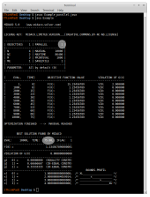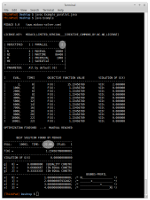# MIDACO Parallelization in Java (Fork/Join)

Running MIDACO in serial and parallel on a cpu-time expensive objective function.

 Example_parallel.java   Midaco.java  [ parallel version ] Serial Mode (P=1) Parallel Mode (P=2) Parallel Mode (P=4)Total Time: 75 Seconds Total Time: 22 Seconds Total Time: 13 Seconds Original: x1 Speed Up:  x3.4 Speed Up:  x5.7

The results can be reproduced with the limited MIDACO version (available here).

Parallelization in Java requires the java.util.concurrent package to be installed.

Particular the Midaco.java [ parallel version ] will need following imports:

import java.util.concurrent.ForkJoinPool;
import java.util.concurrent.RecursiveAction;

Note on Super-Linear Speedup

Due to the highly efficient parallelism scheme implemented in MIDACO's Java gateway the parallelization benefit exhibits an even super-linear speedup. This means for example: If a parallelization factor P=2  is used, an actual speedup of 3.4 times is achieved (instead of only 2 times). Super-linear speedups are rare and very pleasant. More information on super-linear speedups can be found for example here: https://en.wikipedia.org/wiki/Speedup#Super-linear_speedup